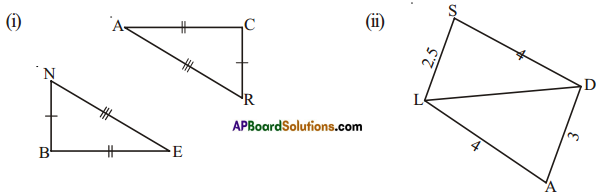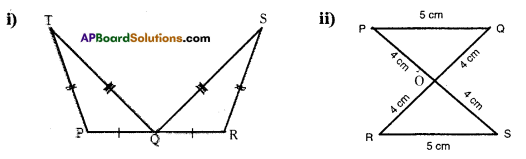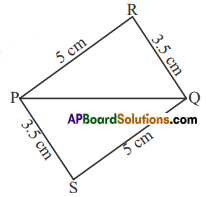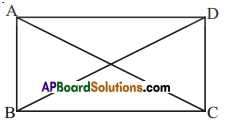# AP Board 7th Class Maths Solutions Chapter 8 Congruency of Triangles Ex 1

AP State Syllabus AP Board 7th Class Maths Solutions Chapter 8 Congruency of Triangles Ex 1 Textbook Questions and Answers.

## AP State Syllabus 7th Class Maths Solutions 8th Lesson Congruency of Triangles Exercise 1Question 1.
Decide whether the SSS congruence is true with the following figures. Give reasonsSolution:
i) From the figures,
BE = CA
EN = AR
BN = CR
∴ S.S.S congruency is true.

ii) From the figure,
AL = SD = 4 cm
LD = LD = common side
AD ≠ LS (3 cm ≠ 2.5 cm)
∴ S.S.S congruency is not ture.Question 2.
For the following congruent triangles, and the pairs of corresponding angles.Solution:
i) ∠P = ∠R
∠T = ∠S
∠TQP = ∠SQR

ii) ∠P=∠S
∠Q = ∠R
∠POQ = ∠SOR

Question 3.
(i) ΔPQR ≅ ΔPQS
(ii) ΔPQR ≅ ΔQPS
(iii) ΔPQR ≅ ΔSQP
(iv) ΔPQR ≅ ΔSPQSolution:
(ii) ΔPQR ≅ ΔQPS is correct.Question 4.
In the figure given below, AB = DC and AC = DB. Is ΔABC ≅ ΔDCB.Solution:
Given that AB = DC
AC = DB
Also from the figure ∠B = ∠C
∴ ΔABC ≅ ΔDCB